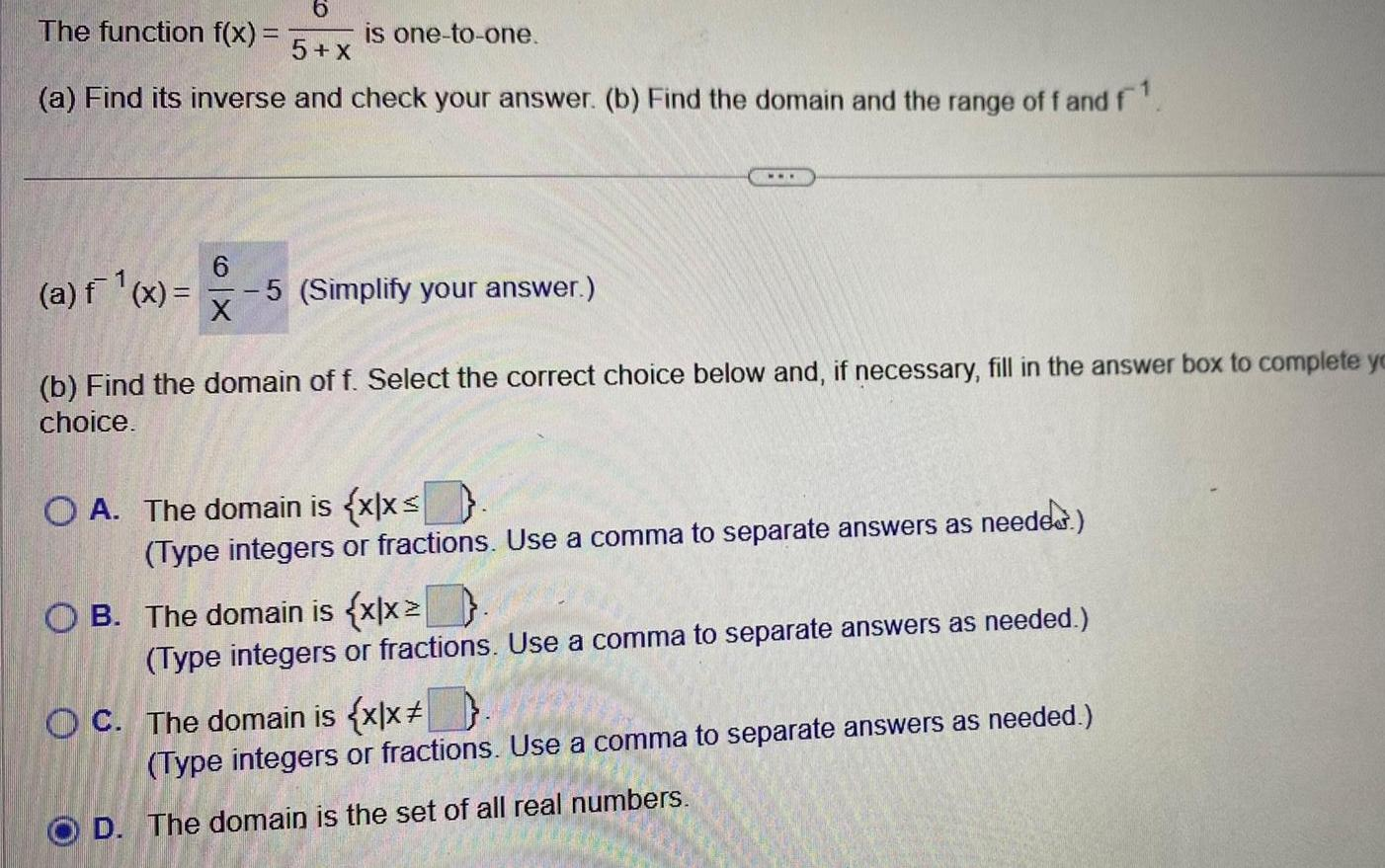Question:

# 6 The function f x is one to one 5 X a Find its inverse and

Last updated: 11/20/20236 The function f x is one to one 5 X a Find its inverse and check your answer b Find the domain and the range of f and f a f x 5 Simplify your answer 6 X b Find the domain of f Select the correct choice below and if necessary fill in the answer box to complete yo choice O A The domain is x x Type integers or fractions Use a comma to separate answers as needed OB The domain is x x Type integers or fractions Use a comma to separate answers as needed OC The domain is x x Type integers or fractions Use a comma to separate answers as needed D The domain is the set of all real numbers